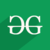Related Articles
Wand resize() function in Python
• Last Updated : 10 May, 2020

Resize image refers to change dimensions of original image in order to convert original image in dimensions that are perfect to use.Scaling Down refers to decrease dimensions of images and make image size smaller. While Scaling Up refers to increase dimensions of an image, and making image size larger.
resize() function is used in order to Resize an image.

Synatx:

```wand.image.resize(width=None, height=None, filter='undefined', blur=1)
```

Parameters :

Parameter Input Type Description
width numbers.Integral New width of image
height numbers.Integral New height of image
filter basestring or numbers.Integral A filter type to use for resizing.
blur numbers.Real The blur factor where > 1 is blurry, < 1 is sharp

Example 1 :

Input Image :`# import Image from wand.image ` `from` `wand.image ``import` `Image ` ` `  `# read image using Image() function ` `with Image(filename ``=` `'gog.png'``) as img: ` `    ``# resize image using resize() function ` `    ``img.resize(``50``, ``50``, ``filter` `=` `'undefined, blur ``=` `1``) ` ` `  `    ``# save resized image ` `    ``img.save(filename ``=` `'resized_gog.png'``) `

Output :Example 2 :

Input Image :
Input will be from a url.GeeksforGeeks

 `# import required libraries ` `import` `urllib3 ` `from` `cStringIO ``import` `StringIO ` `from` `wand.image ``import` `Image ` `from` `wand.display ``import` `display ` ` `  `# load image from url ` `http ``=` `urllib3.PoolManager() ` `r ``=` `http.request(``'GET'``, ``'https://media.geeksforgeeks.org/wp-content/uploads/geeksforgeeks-6.png'``) ` `f ``=` `StringIO(r.data) ` ` `  `# read image using Image() function ` `with Image(``file``=``f) as img: ` ` `  `    ``# resize image using resize() function ` `    ``img.resize(``400``, ``300``) ` ` `  `    ``# save image  ` `    ``img.save(filename ``=` `'gogurl.png'``) ` ` `  `    ``# display final image ` `    ``display(img) `

Output :Attention geek! Strengthen your foundations with the Python Programming Foundation Course and learn the basics.

To begin with, your interview preparations Enhance your Data Structures concepts with the Python DS Course.

My Personal Notes arrow_drop_up
Recommended Articles
Page :